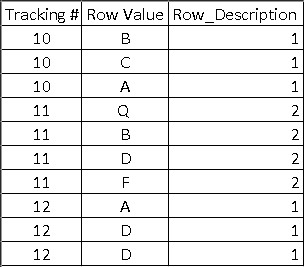# TSQL - Assign same value to every line where condition is met for one line using case logic

I'm looking to find a cleaner way of doing this. I'm looking to find all of the tracking numbers where one line meets a condition and then assign a value to every row of that tracking number. I'm currently doing this with case logic but only get halfway there without creating more statements. Way to do this cleanly in one?

End result would look like:The sql below only gives me the the case value for the line where I'd want it for every line in the tracking number where it applied.

select [Tracking Number] ,
CASE WHEN [Tracking Number] in
(Select distinct [Tracking Number] where ([Row Description] like '%A%') Then '1'
WHEN [Tracking Number] in
(Select distinct [Tracking Number] where ([Row Description] like '%B%') Then '2'
ELSE '' END as Row_Description
from dwh.mytable

Thanks!

``````
;with cte_calc_Row_Values as (
select [Tracking Number] ,
max(CASE WHEN [Row Description] like '%A%' Then '1'
WHEN [Row Description] like '%B%' Then '2'
WHEN [Row Description] like '%C%' Then '3' end) as [Row Value]
from dwh.mytable
GROUP BY [Tracking Number]
)
select a.[Tracking Number], b.[Row Value], a.[Row Description]
from dwh.mytable a
left outer join cte_calc_Row_Values b ON b.[Tracking Number] = a.[Tracking Number]
``````